# Chain condition

(diff) ← Older revision | Latest revision (diff) | Newer revision → (diff)

A finiteness condition for ascending or descending chains in a partially ordered set. The descending chain condition for a partially ordered set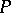states: For any chain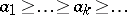of elements there is a numbersuch that. This condition is equivalent to each of the following properties of:

1) every non-empty subsethas at least one minimal element in(the minimum condition);

2) all elements ofhave a given propertyif all minimal elements ofhave this property and if the validity offor any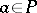can be deduced from the fact thatis valid for all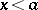,(the inductiveness condition).

The inductiveness condition enables one to carry out proofs and constructions by induction for sets with the descending chain condition. Here ifis totally ordered (and hence well-ordered) one obtains transfinite induction, and ifis isomorphic to the set of natural numbers, one obtains ordinary mathematical induction (see Induction axiom).

The ascending chain condition (and assertions equivalent to it) is formulated in a dual way (see Duality principle in partially ordered sets); it therefore states that if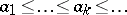is an ascending chain in a partially ordered set, then forlarge enough. In a lattice with the ascending chain condition every ideal is principal. Every finite set obviously satisfies both chain conditions, but the converse assertion (that a set satisfying both these conditions is finite) is false. A lattice satisfying the descending and ascending chain conditions is complete.

In algebra, chain conditions are mainly applied to sets of subsystems of various algebraic systems ordered by inclusion (see for example, Artinian module; Artinian group; Artinian ring; Composition sequence; Noetherian module; Noetherian group; Noetherian ring).

How to Cite This Entry:
Chain condition. Encyclopedia of Mathematics. URL: http://encyclopediaofmath.org/index.php?title=Chain_condition&oldid=15683
This article was adapted from an original article by T.S. Fofanova (originator), which appeared in Encyclopedia of Mathematics - ISBN 1402006098. See original article